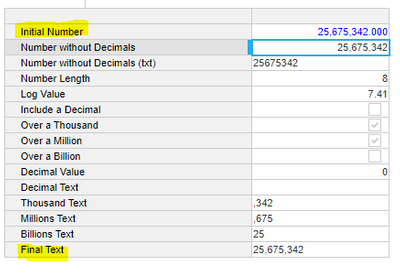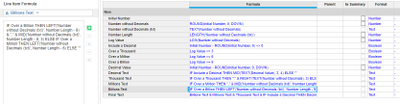# Convert Text to Number following comma formatHello,

Is there an easy way i can convert number to text format but still following the number format for comma?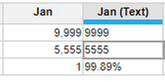Tom

Tagged:

•Hi Tom,

It is quite painful to do, but you can achieve this with IF statement loop and length check constraints such as:
IF LENGTH(TEXT(Number)) > 6 THEN LEFT(TEXT(Number), LENGTH(TEXT(Number)) - 6) & "," & MID(TEXT(Number), LENGTH(TEXT(Number)) - 5, 3) & "," & RIGHT(TEXT(Number), 3) ELSE IF <Write similar condition for greater than 3 digits> ELSE TEXT(Number)

TEXT functions as well as text formatted line items are always best to avoid so do try avoiding this if possible. Another thing if you want to optimize the formula above, is to reference the TEXT(Number) conversion and the LENGTH(TEXT(Number)) instead of calculating that in the formula each time

Let me know if you need additional help

Regards,
Anirudh

•Hi,

The first solution I would suggest is to try and convert your data at the source from text to number or map it to a number format on import. We should try and minimise data transformation of this type within the platform if the solution can be found in converting the source data before importing into Anaplan.

If this is not possible the following solution my help.

The issue here is that we need to remove the comma from the text string. Depending on the size of text we need to cycle through a series of data transformations to isolate the text we wish to keep and to then reassemble the text string before we convert it to a number.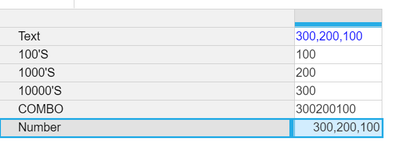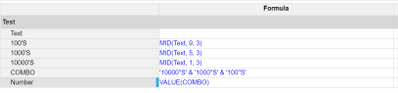This does not take account of decimals as your example did not include them.

If this was require you could adjust the '100;s' mid formula to extend further to capture all the data to the right of the decimal.

•Hi @ChrisAHeathcote , Thanks for responding. In your example, you are converting text to number, what we need is to convert is to convert the number to text format but still following the number format for comma.

We are doing this because we have a case where the line item composes of mix format, absolute and percentage number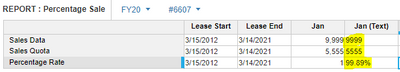.

•If we tweak the line items or formulas slightly we can achieve what you are looking for;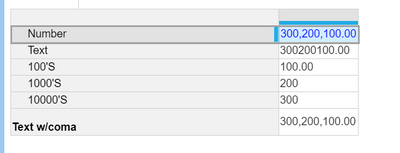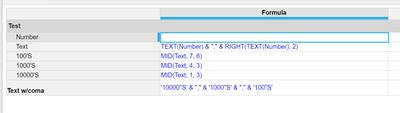•I would avoid placing all the steps within a single function as this does not follow best practice and will likely cause performance issues if you have a large list to transform. Use a systems module to transform your data and then reference this in your target module if you do not want to clutter your target module with too many line items.

However, both approaches outlined above follow the same principle that you need to query the length of the string so that you can target correctly where you place the comma in the final output.
•Yes completely agree, I've mentioned breaking up into references in my reply!
•Hi Tom,

Here is one way to do it. Only limitation: you'd have to create additional loops for numbers higher then 999 billions

I hope this helps.

Regards